## Posts

### Understanding Geometric Nonlinearity - 1 by Kotur Raghavan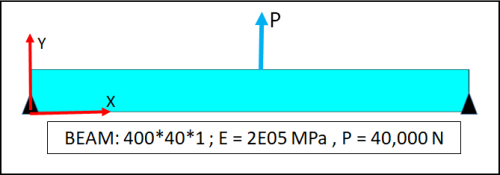Preamble Any structure deforms when subjected to loads. The basic question is whether the deformations affect the structural behavior to any practically significant extent. If they do, we know that the small deformation theories are inadequate and it is necessary to invoke large deformation formulation to obtain acceptable results. In other words, one has to invoke the  “Geometric Nonlinearity” option while using FEA packages to obtain good results. The general thumb-rule is that when the deformations are comparable to the dimensions of the structure, the effects of geometric nonlinearity become noticeable. In the small deformation theory or linear elasticity, the basic assumption is that the displacements in orthogonal directions are uncoupled. In Cartesian geometry, the displacement fields X- and Y- directions are independent of one another. In the cylindrical coordinate system, the displacement fields in the radial and tangential directions are assumed to be independent of one

### Minimum Constraints in FEA – 5. Submodeling by Kotur Raghavan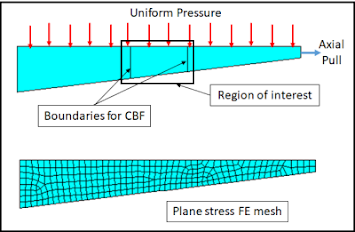General Submodeling is a technique usually adopted in order to fine-tune the stress results at critical locations. One conducts local analysis with refined mesh by imposing the boundary conditions from the full or global model. Deformations from the full (coarse) model are interpolated with the help of element shape functions. This is the conventional approach and is based on Saint-Venant’s principle. This can be called as Cut-Boundary-Displacement (CBD) approach. The approach is well established and promotes productivity enhancements as the need to refine the mesh in the entire structure is eliminated. However, there are two limitations. The first one is that the geometry should be identically similar in the global and the submodel. If there is any change in stiffness the computed stresses will be in error.  In addition, it is valid strictly for linear analysis. The above limitations can be overcome by resorting to one of the two variants. The first alternative is based on impos

### Minimum Constraints in FEA – 4. Inertia Relief Application by Kotur Raghavan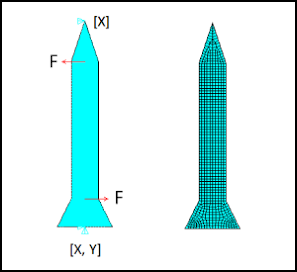General Many analysis packages have provision for applying “inertia relief”. This is a highly specialized operation which is applicable to objects in flight. Objects in flight such as rockets are always in dynamic equilibrium. Any manoeuvre load applied to them will be in equilibrium with the inertia forces. Stress analysis of an aircraft or a rocket under the action of manoeuvre loads requires that the loads be applied in the normal way and that the inertia relief operation be invoked. The programs internally computes inertia forces and moments which are equal and opposite to the applied loads. An important requirement is that just the minimum constraints be imposed on the structure. This is demonstrated by way of a simple example problem. The author has experienced that this capability has often been incorrectly used leading to erroneous results. As stated already, the command concerned is for a special application. Example Problem The example is that of a rocket-like objec

### Minimum Constraints in FEA – 3. Zero Resultant Force Problems by Kotur Raghavan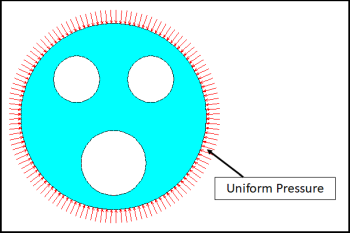General Objects like floating bodies and vehicles in flight have two distinct features. In the first place, they are subject to self-equilibrated system of forces. Secondly, they will have no identifiable displacement constraints. Problems of this class can be solved by applying minimum constraints. This aspect will be demonstrated by way of a few case studies. Perforated Disk under the action of uniform Circumferential Pressure The object shown in Fig. 1 is the first case study problem. Plane stress analysis is carried out. Fig. 1 Two independent analyses are carried out for two different sets of displacement constraints. The constraint sets C1 and C2 are shown in Fig. 2. In each case the rules are adhered to. Fig. 2 The nodes chosen for constraints are arbitrary. We can expect the deformation patterns to be different for the two different constraint sets. This is confirmed by the contours of resultant deformations shown in Fig. 3. Fig. 3 The stresses, howe

### Minimum Constraints in FEA – 2. Sanity Checks by Kotur Raghavan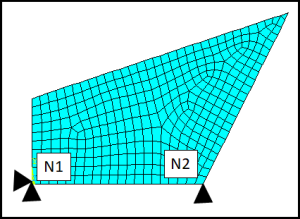General An important property of “minimum constraints” is that they permit free thermal expansion or contraction of the structure when it is subjected to a uniform temperature field. This becomes evident by visual examination of the applied constraints in a 2D problem (Fig. 1).                                                                                  Fig. 1 The node N1 is the fixed point. Free thermal displacement is permitted in the X- direction as there are no other X- direction constraints. The object will freely expand or contract in the Y- direction relative to the line joining N1 to N2. Fig. 2 Similar conditions can be visualized in the case of three-dimensional objects (Fig. 2).   The node N1 is the stationary point. The cube has free thermal flow in the X- direction with respect to N1. Its free thermal flow in the Y- direction is with respect to the line joining N1 to N2. The free expansion in the Z- direction is relative to the XY- plane passing through t

### Minimum Constraints in FEA – 1. Overview by Kotur Raghavan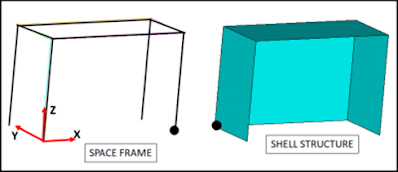Description 1.        In any finite element structural analysis model, minimum number of constraints refer to the constraints needed just to prevent rigid body motion. In a mathematical sense, it is the minimum number of degrees of freedom to be arrested so that the stiffness matrix becomes non-singular so that a solution is possible. 2.        Any object in space has six degrees of freedom. They are three translations and three rotations. Any object in two-dimensional space has three degrees of freedom. They are the two translation and one in-plane rotation. 3.        Therefore it follows that for structures in three-dimensional space, six constraints are needed. Likewise for structures in two-dimensional space, three constraints are needed. 4.        In structural models involving flexural elements, that is beams and shells, all the required constraints can be applied at a single node and a solution will be possible.   Fig. 1   5.        In Fig. 1 above typical structur

### Consistent Units in FEA by Kotur Raghavan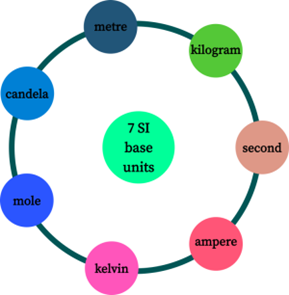General This article can be treated as a refresher of basics related to units and dimensions. It is an obvious need that one should work with consistent units in order to get meaningful results. This is true in any problem associated with the physical world and structural analysis is no exception. Over most of the world metric system is in use. The United States, however, is still sticking to the British system of units. It is necessary that engineers are conversant with both the metric and the British system of units. Fig. 1 Description 1.        In any structural analysis we deal with four basic entities. They are LENGTH, FORCE (or MASS), TIME and TEMPERATURE. All other material properties are derived from these basic ones. 2.        In British (or imperial) system INCH is the unit used for length. In SI (abbreviation of Système international) system   meter  (m) is the standard unit. Due to the design office and shop floor preference,  millimetre  (mm) has also become an